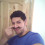WHAT'S NEW?

# Parameters and Arguments

Parameters

Parameters are something which is enclosed inside parenthesis of a method.

For example:

public void square(int num)

Text marked in green color are the parenthesis of method square(),
hence int num is the parameter.

So simply, ANYTHING WITHIN THE PARENTHESIS OF A METHOD IS PARAMETER.

Arguments

Arguments are the values passed to the parameter.

For example:

obj.square(5);

Hence 5 is argument passed to the parameter of the method we discussed earlier in parameters.

Arguments can be any of the stated below.

1) Constant

obj.square(5);

This one is simple to understand. We can pass directly a value which is a constant.

2) Variable

int z=25;
obj.square(z);

We can make a variable and pass it as an argument.
It is like wrapping the constant in a variable and passing it.

3) Expression

int i=2;
int j=4;

obj.square((i+j));

Expression is collection of operator(+,-,*,/,%) and operands (here i and j).

Hence an expression will be first executed and then output of expression will be passed as argument.

So here first i+j will be executed that will give the output 6 and it will be passed as an argument.

4)Method returning some value

public int divideHalf(int num)
{
int ans=num/2;
return ans;
}

obj.square(divideHalf(30));

Only those method which returns some value and of course those values should match with the parameter can be passed as an argument.

If method returning some value are passed then the method is executed first, so here divideHalf() will get executed first and yield an output 15, and it will be passed as an argument to square()

1.